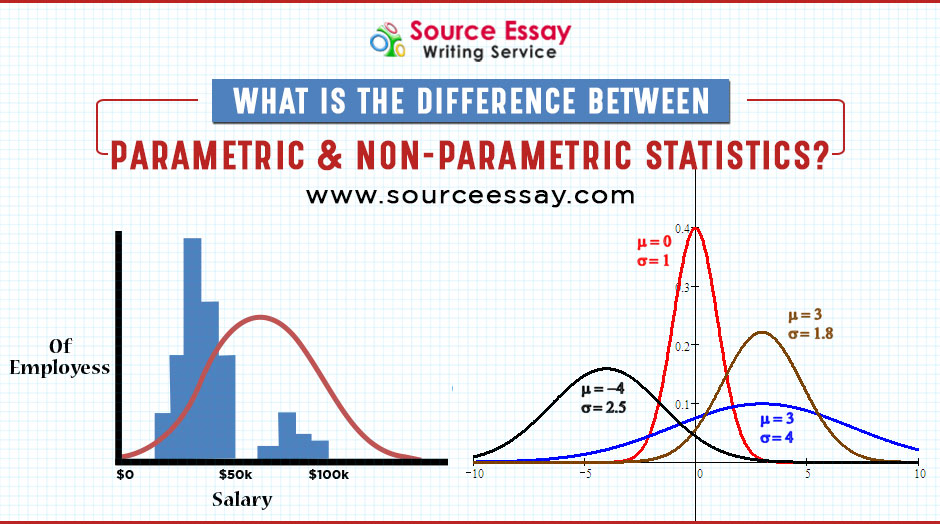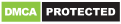# What Is The Difference Between Parametric And Non-Parametric Statistics?Share

A hypothesis is constructed by social researches where an assumption is made based on certain generalized rules applied to a population.

The hypotheses are further tested by using tests that can be either parametric or non-parametric. Parametric tests are standard tests that are common and studied earlier to non-parametric tests when a research is being performed.

Students can avail statistics assignment help for any kind of assistance in their assignments related to statistics. In this article we are going to discuss the difference between parametric and non-parametric statistics.

### Parametric Statistics

• In case of parametric test, the process of performing a test is relatively simple. A hypothesis is constructed based on assumption that a particular law can be applied to a population.
• Based on hypothesis a test is conducted and data gathered is analyzed statistically.
• Data collected is usually represented as a graph and the hypotheses law is considered to be the mean value of that particular data.
• A parametric test is used where there are clear assumptions about the population and there is a lot of information available on it.
• Parametric test is a test where data is collected using parametric statistics. There are online essay writers available for students. Where students get all the guidance required to solve assignments on parametric statistics.

### Non-Parametric Statistics

• A non- parametric does not make any assumptions and the central tendency is measured with the median value.
• In case of non-parametric distribution of population is not required which are specified using different parameters.
• It is not based on the underlying hypothesis rather it is more based on the differences of the median.
• The method of test used in non-parametric is known as distribution-free test.
• The test variables are based on the ordinal or nominal level.

Students can seek the help from assignment writers to solve assignments on non-parametric statistics.

### Key  Differences  Between  Parametric  And  Non-Parametric  Statistics

• The parametric tests are based on assumptions using the data connected to the normal distribution used in the analysis. Knowledge on the parameters is very essential. Whereas on the other hand non-parametric test does not depend on any parameters.
• It does not work on assumptions.
• The analysis that is derived from data in the case of parametric test is called probabilistic distribution. On the other hand, in case of non-parametric the probability distribution is arbitrary and their central tendency can’t be measured.
• In case of parametric test data is measured using mean value where as in non- parametric the median value s used.
• The information used varies in both the cases. In case of parametric certain knowledge on population is important because of parameters related to population whereas in non-parametric no previous knowledge on population is needed.
• A parametric test is based on fixed parameters, previous knowledge, and assumptions whereas the non-parametric statistics is done arbitrarily on independent variables.
• In case of parametric statistics derived is based on distribution whereas in case of non-parametric statistics is not based on any kind of distribution.
• The measurement of variable of interest in the case of parametric is done on interval or ratio level whereas on the other hand nominal of interest is measured using nominal or ordinal scale in non-parametric.
• Unlike parametric test there is no requirement of information on population in non-parametric.
• Parameter test is applicable to only for variables whereas non-parametric test is applicable both for variables and attributes.
• Person’s coefficient or co-relation is used to measure degree of association between two different variables in case of parametric tests whereas spearman’s rank correlation is used in non-parametric tests.

There is essay writing help available for students to help them in submitting their assignments on time.

### Conclusion

In simpler terms it can be said that while performing a particular research if the information about the population is available in the form of parameters, then in that case, parametric statistics can be used.And, the test conducted using this statistical analysis is called parametric test.

Whereas, if the hypothesis on population needs to be tested without the availability of knowledge on population, then in that case,non- parametric statistics is used. And the test that is done analyzing the non-parametric statistical report is called non-parametric test.

We can sum-up the differences between parametric and non-parametric differences in the following points-

• In case of parametric assumptions are made. In case of Non-parametric assumptions are not made.
• The value for central tendency is mean value in parametric statistics whereas it is measured using the median value in non-parametric statistics.
• The correlation in parametric statistics is Pearson whereas, the correlation in non-parametric is Spearman.
• The probabilistic distribution is normal in parametric and arbitrary in non-parametric.
• The knowledge on population is required in parametric and not required in non-parametric.
• Parametric statistics is used for interval data and non-parametric statistics is used for nominal data.
• Parametric statistics is applicable for variables and non-parametric statistics is applicable for both attributes and variables.
• T- Test, Z-Test are examples of parametric whereas, Kruskal-Wallis, Mann- Whitney are examples of no-parametric statistics.

Students might find it difficult to write assignments on parametric and non-parametric statistic. But catering to the requirements of students SourceEssay has come up with online assignment help to aid students in writing assignments related to parametric non-parametric statistics.

They have a team of experts who have years of experience in solving these equations.

They not only write assignments for the students but also assignments checked by a team of expert proof readers and editors before they are delivered back to the students. The writers are highly professional and committed in their work. They make sure the assignments are non-plagiarized. They ensure they deliver high-quality assignments which are at par with the quality standards of the university and the students never miss their deadlines.

In case of any doubt’s students are given 24*7 round the clock customer support where they are guided by experts.

These services have made the task of students much easier and taken the assignment pressure off their shoulders.Hello! Welcome to Sourceessay.